# pymcmcstat.procedures package¶

## pymcmcstat.procedures.CovarianceProcedures module¶

Created on Thu Jan 18 07:55:46 2018

Description: Support methods for initializing and updating the covariance matrix. Additional routines associated with Cholesky Decomposition.

@author: prmiles

class pymcmcstat.procedures.CovarianceProcedures.CovarianceProcedures[source]

Bases: object

Covariance matrix variables and methods.

Attributes:
display_covariance_settings(print_these=None)[source]

Display subset of the covariance settings.

Args:
• print_these (list): List of strings corresponding to keywords. Default below.

print_these = ['qcov', 'R', 'RDR', 'invR', 'last_index_since_adaptation', 'covchain']

setup_covariance_matrix(qcov, thetasig, value)[source]

Initialize covariance matrix.

If no proposal covariance matrix is provided, then the default is generated by squaring 5% of the initial value. This yields a diagonal covariance matrix.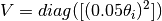If the initial value was one, this would lead to zero variance. In those instances the variance is set equal to qcov[qcov==0] = 1.0.

Args:

## pymcmcstat.procedures.ErrorVarianceEstimator module¶

Created on Thu Jan 18 13:12:50 2018

@author: prmiles

class pymcmcstat.procedures.ErrorVarianceEstimator.ErrorVarianceEstimator[source]

Bases: object

Estimate observation errors.

Attributes:
gammar(m, n, a, b=1)[source]

Random deviates from gamma distribution.

Returns a m x n matrix of random deviates from the Gamma

distribution with shape parameter A and scale parameter B: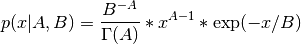Args:
gammar_mt(m, n, a, b=1)[source]

Wrapper routine for calculating random deviates from gamma distribution using method of Marsaglia and Tsang (2000) [MT00].

Args:
update_error_variance(sos, model)[source]

Update observation error variance.

Strategy: Treat error variance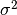as parameter to be sampled.

Definition: The property that the prior and posterior distributions have the same parametric form is termed conjugacy.

Starting from the likelihood function, it can be shown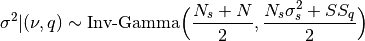where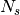and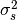are shape and scaling parameters,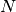is the number of observations, and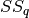is the sum-of-squares error. For more details regarding the interpretation ofand, please refer to [Smi14] page 163.

Note

The variablesandcorrespond to N0 and S20 in the ModelSettings class, respectively.

Args:

## pymcmcstat.procedures.PriorFunction module¶

Created on Thu Jan 18 09:10:21 2018

Description: Prior function

@author: prmiles

class pymcmcstat.procedures.PriorFunction.PriorFunction(priorfun=None, mu=array(), sigma=array([inf]))[source]

Bases: object

Prior distribution functions.

Attributes:
classmethod default_priorfun(theta, mu, sigma)[source]

Default prior function - Gaussian.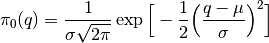Args:
evaluate_prior(theta)[source]

Evaluate the prior function.

Args:

## pymcmcstat.procedures.SumOfSquares module¶

Created on Wed Jan 17 16:21:48 2018

@author: prmiles

class pymcmcstat.procedures.SumOfSquares.SumOfSquares(model, data, parameters)[source]

Bases: object

Sum-of-squares function evaluation.

Description: Sum-of-squares (sos) class intended for used in MCMC simulator. Each instance will contain the sos function. If the user did not specify a sos-function, then the user supplied model function will be used in the default mcmc sos-function.

Attributes:
evaluate_sos_function(theta, custom=None)[source]

Evaluate sum-of-squares function.

Args:
Returns:
classmethod mcmc_sos_function(theta, data, nbatch, model_function)[source]

Default sum-of-squares function.

Note

This method requires specifying a model function instead of a sum of squares function. Not recommended for most applications.

Basic formulation: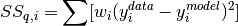where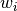is the weight of a particular data set, and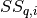is the sum-of-squares error for the i-th data set.

Args:
Returns: